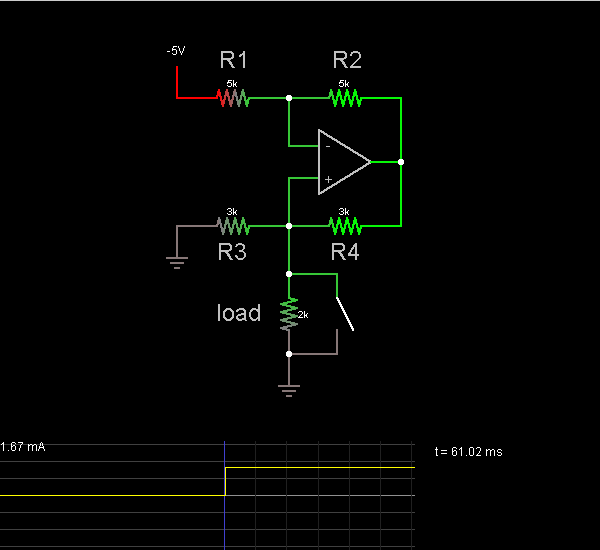# Circuit Simulator - Howland Current Source

## Where can I get Howland Current Source Circuit Diagram with Explanation?

IndiaBIX provides you lots of fully solved Howland Current Source circuit diagram with detailed explanation and working principles.

## How to design a Howland Current Source (electronic circuit)?

You can easily design the Howland Current Source circuit by practicing the exercises given below. Here you can design and simulate your own electronic circuits with this Online Circuit Designer and Simulator.### Circuit Description:

This circuit shows a current source. The current through the load is the same regardless of the position of the switch.

The op-amp attempts to keep the voltages at both terminals the same, so V = V+. Call V1 the voltage across R1, and I1 the current across R1. Then V2 = V4. Since I1 = I2 and R1 = R2, V1 = V2 = V4.

V = V+ = V1 - 5V

I3 = V+/R3 = (V1 - 5V)/R3.

I4 = V4/R4 = V1/R3.

V1/R3 = (V1 - 5V)/R3 + Iload

Iload = 5V / R3 = 1.67 mA -- Credits: Mr. Paul Falstad.

 Nick said: (Jan 27, 2012) That's Widlar's current source. Still nicely done.

 Tom said: (Jan 4, 2013) Why does the current stop in resistor R3 when the switch is closed? Is it just so small that it does not show? There should be some current unless these are theoretical Zero resistance switch and conductors ("wires").

 Someone said: (Apr 17, 2013) It stops because when you flip the switch, you created a path where resistance equals 0.

 S_Eng said: (Apr 15, 2014) The demonstration circuit is flawed, and contrary to the text statement, and as drawn, the current in the load WILL vary. It would be better to show an additional load switched in, in series with the first load, thus showing that the circuit delivers a constant value current despite the changing load.

 Spf said: (Nov 5, 2014) Sorry, doesn't work. Did you actually try it on SPICE?